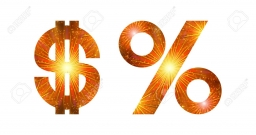# Increase 7409

If you increase the unknown number by 14 percent, you get the number 136.8. Find an unknown number.

x =  120

### Step-by-step explanation:

x + x · 14/100 = 136.8

114x = 13680

x = 13680/114 = 120

x = 120

Our simple equation calculator calculates it.Did you find an error or inaccuracy? Feel free to write us. Thank you!

Tips for related online calculators